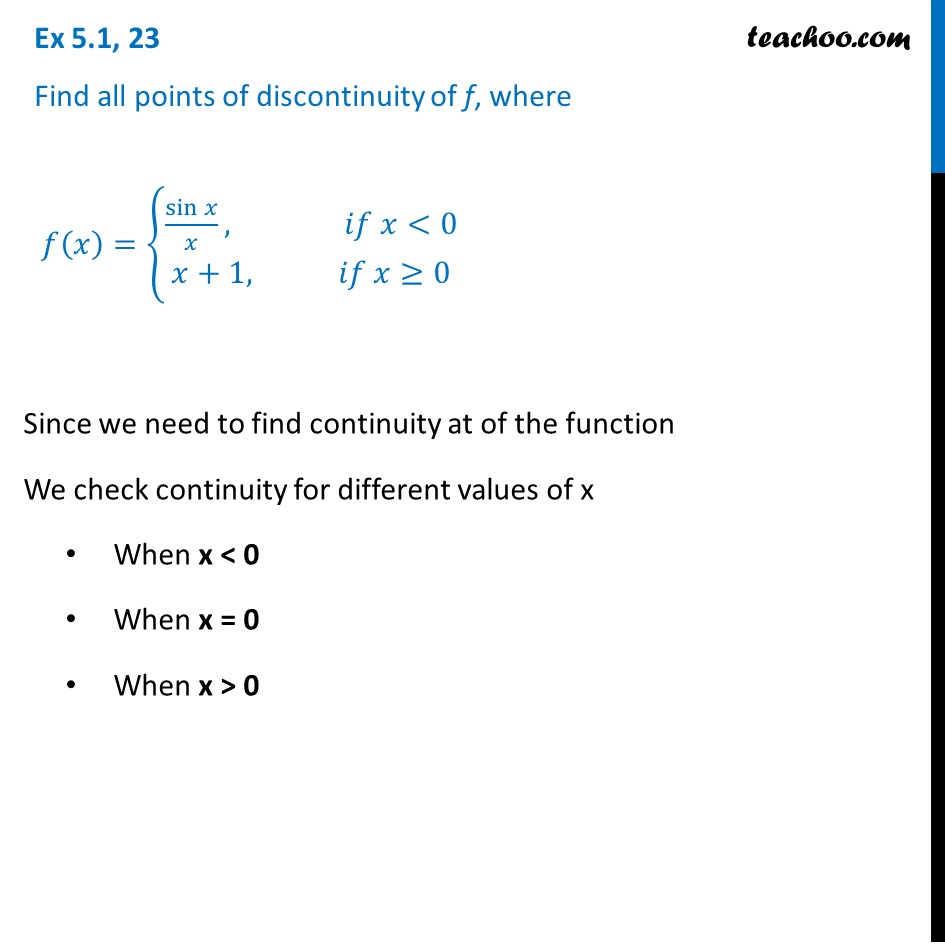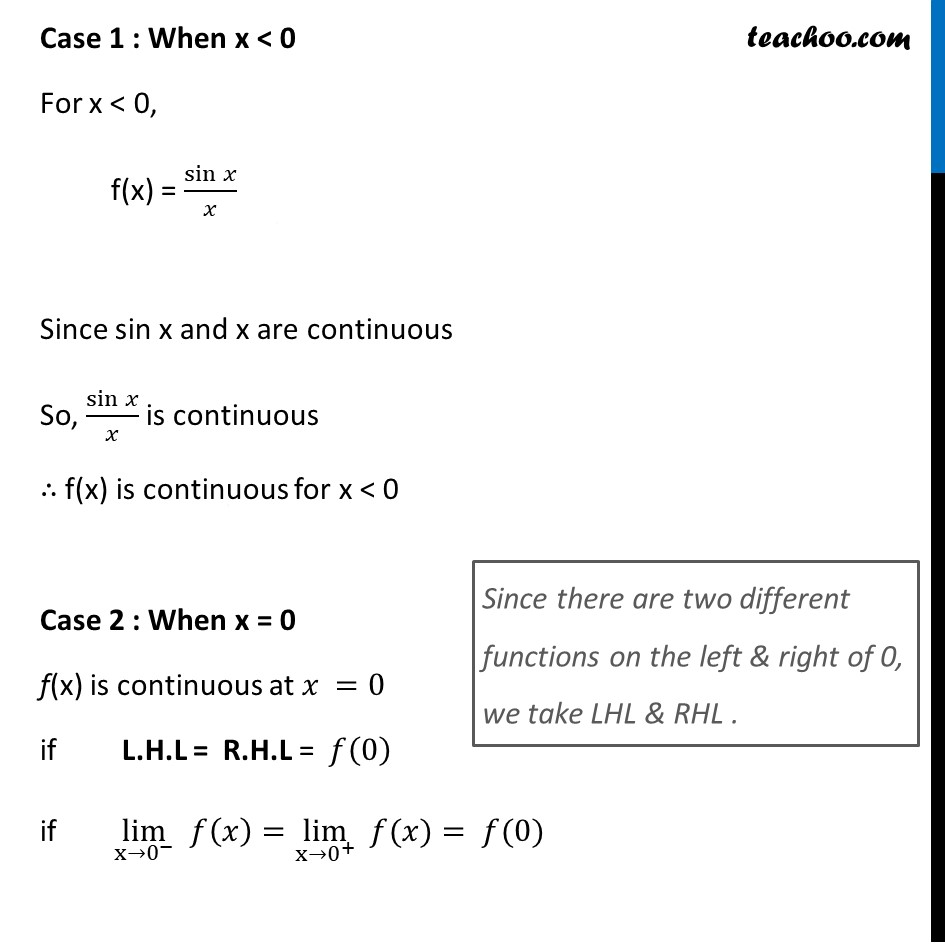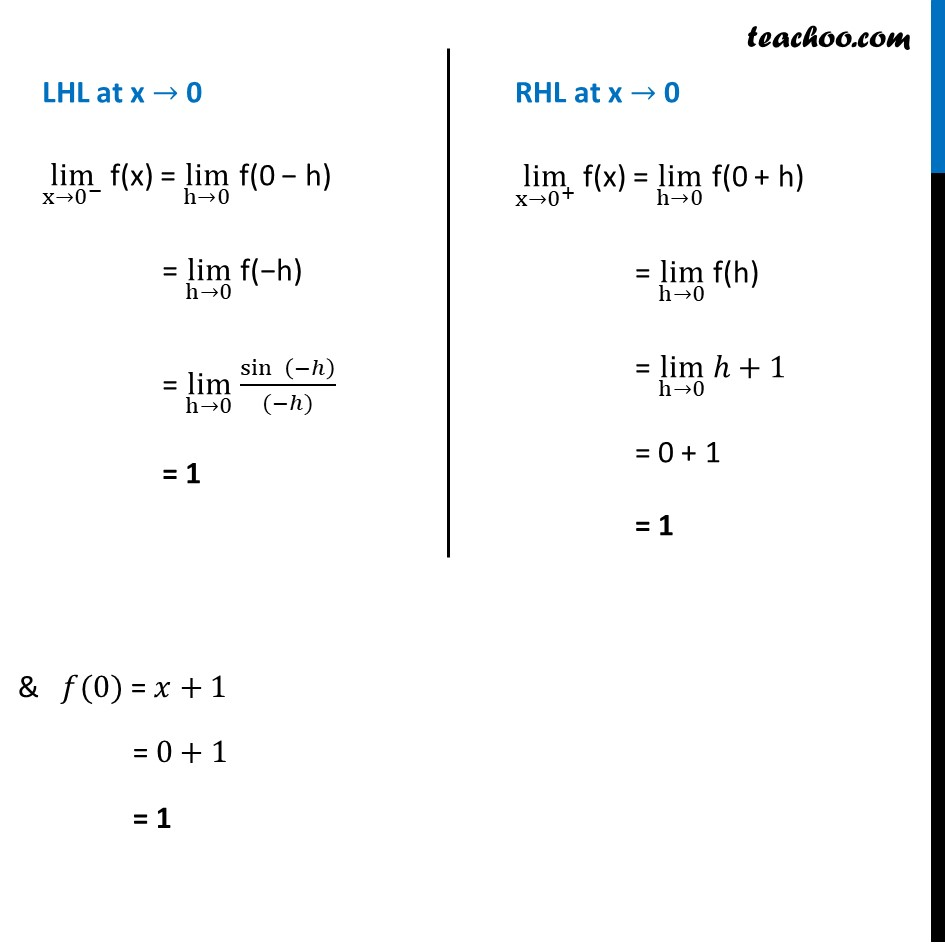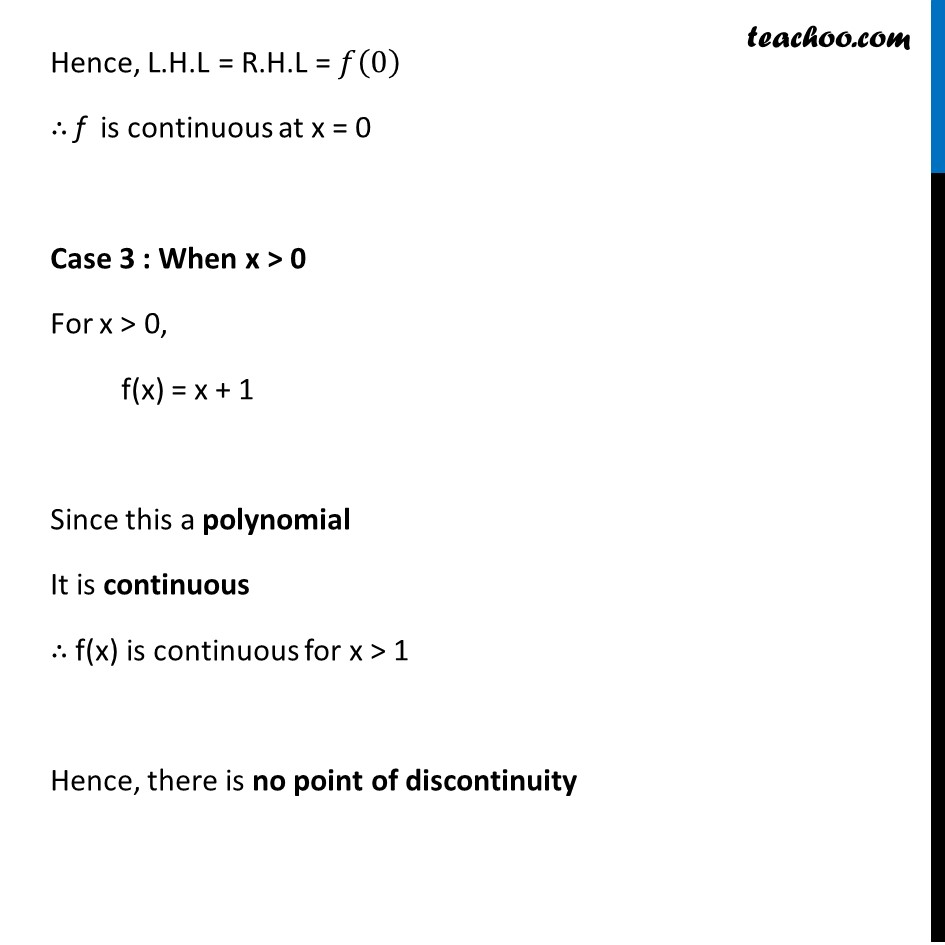Ex 5.1

Chapter 5 Class 12 Continuity and Differentiability
Serial order wiseLearn in your speed, with individual attention - Teachoo Maths 1-on-1 Class

### Transcript

Ex 5.1, 23 Find all points of discontinuity of f, where 𝑓(𝑥)={█(sin⁡𝑥/𝑥, 𝑖𝑓 𝑥<0@&𝑥+1, 𝑖𝑓 𝑥≥0)┤ Since we need to find continuity at of the function We check continuity for different values of x When x < 0 When x = 0 When x > 0 Case 1 : When x < 0 For x < 0, f(x) = sin⁡𝑥/𝑥 Since sin x and x are continuous So, sin⁡𝑥/𝑥 is continuous ∴ f(x) is continuous for x < 0 Case 2 : When x = 0 f(x) is continuous at 𝑥 =0 if L.H.L = R.H.L = 𝑓(0) if lim┬(x→0^− ) 𝑓(𝑥)=lim┬(x→0^+ ) " " 𝑓(𝑥)= 𝑓(0) Since there are two different functions on the left & right of 0, we take LHL & RHL . LHL at x → 0 lim┬(x→0^− ) f(x) = lim┬(h→0) f(0 − h) = lim┬(h→0) f(−h) = lim┬(h→0) sin⁡〖 (−ℎ)〗/((−ℎ)) = 1 RHL at x → 0 lim┬(x→0^+ ) f(x) = lim┬(h→0) f(0 + h) = lim┬(h→0) f(h) = lim┬(h→0) ℎ+1 = 0 + 1 = 1 & 𝑓(0) = 𝑥+1 = 0+1 = 1 Hence, L.H.L = R.H.L = 𝑓(0) ∴ f is continuous at x = 0 Case 3 : When x > 0 For x > 0, f(x) = x + 1 Since this a polynomial It is continuous ∴ f(x) is continuous for x > 1 Hence, there is no point of discontinuity